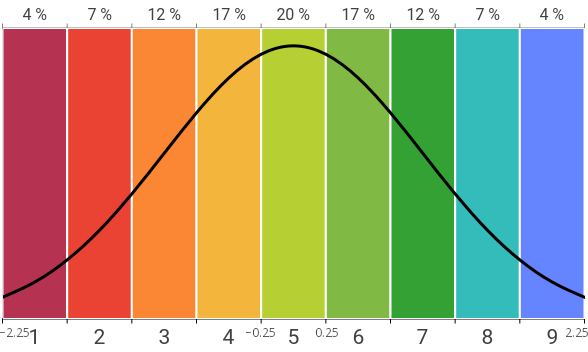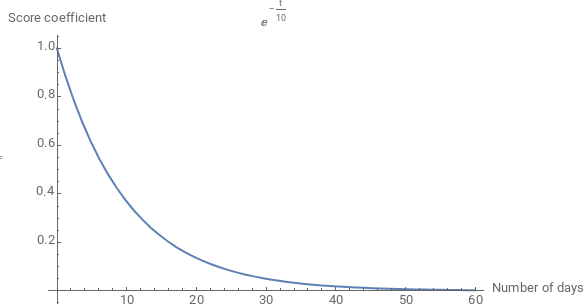# Stanine Ranking System

Just like the day of the exam, EPLtest ranks you against the other candidates with the Stanine system. This Stanine ("STAndard NINE") ranking method would have been used first for the U.S. Army Air Forces psychometric tests, during World War II.

The interpretation is simple: the higher your class is, the better you are compared to the other candidates.

## How does it work?

The Stanine ranking consists in ranking the scores according to a standard normal distribution. To materialize the Stanine classes, we divide the distribution into 9 intervals of width 0.5 standard deviation, except the tails (1 and 9 classes) which have an infinite width.Illustration of the division into 9 classes of 0.5 standard deviation width.
Stanine class Standard deviation range Percent of the best candidates Description
1 [−∞; −1.75] from 0 % to 4 % Very poor
2 [−1.75; −1.25] from 4 % to 11 % Poor
3 [−1.25; −0.75] from 11 % to 23 % Considerably below average
4 [−0.75; −0.25] from 23 % to 40 % Slightly below average
5 [−0.25; 0.25] from 40 % to 60 % Average
6 [0.25; 0.75] from 60 % to 77 % Slightly above average
7 [0.75; 1.25] from 77 % to 89 % Considerably above average
8 [1.25; 1.75] from 89 % to 96 % Superior
9 [1.75; +∞] from 96 % to 100 % Very superior

• People from the class 1 are the 4 % worst candidates.
• People from the class 8 are better than 89 % to 96 % of the candidates.

Most exams require a class of 5 (Average) or 6 (Slightly above average) in all the evaluated abilities, meaning that they eliminate the 40 % worst candidates. Although these numbers depend on how many candidates the selection want to keep, we recommend you to obtain a class of 5 (Average) in all abilities.

## Float classes

For more precision, the EPLtest algorithm is capable of giving you float Stanine classes. Your integer class is calculated by rounding your float class.

For instance, an integer class of 5 corresponds to a float class between 4.5 and 5.5, a class of 7 to a float class between 6.5 and 7.5, and so on.

Note: the ability to see your float class instead of your integer class depends on the subscription you choose.

## Extreme classes (rare)

EPLtest may give a 1− or 9+ Stanine class. It is the same as a 1 or 9 class at the exam.

Theoretically, the class 1 standard deviation ranges from −∞ to −1.75. A 1− class means that, if there were other classes below the 1 class, like a 0 class ranging from −∞ to −2.25 standard deviations, you would be ranked in these classes. The same applies for 9+. It means that the standard deviation of your score is above 2.25, far in the superior tail of the curve.

## How are your averages calculated?The coefficient of your score according to the number of days since you completed the test.

Your average score per test is calculated by an exponential moving average (EMA) of characteristic period 10 days. It has the advantage to favor your recent results over the old ones. If you have no recent result, it will nicely fallback to your old ones.

Age in days 0 Score coefficient Contribution to your average 1.0 26 % 0.97 26 % 0.74 20 % 0.74 20 % 0.25 7 % 0.05 1 % 3.75 100 %